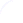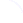Search
 Submission Procedure share: |available in:   PDF (220 kB) PS (89 kB)

get:
 Similar Docs BibTeX Write a comment
get:

DOI:   10.3217/jucs-002-02-0058

### Curve Fitting and Interpolation of Biological Data Under Uncertainties

Svetoslav M. Markov (Research Group for Mathematical Modelling in Biology, Institute of Biophysics, Bulgarian Academy of Sciences, Bulgaria)

Y. Akyildiz (Mathematics Department, Bosphorus University, Turkey)

Abstract: This paper is devoted to the software implementation of two mathematical methods which are often used in biological applications: interpolation and curve fitting in the presence of uncertainties in the input data given in the form of intervals. The methods involve model functions linear in their parameters and are formulated by means of simple expressions in terms of interval arithmetic allowing the computation of verified bounds for the interpolating/approximating functions. The methods are demonstrated for certain classes of nonlinear modelling functions finding applications in biology. A case study involving enzyme-catalysed reaction is considered. The numerical results are performed in the computer algebra system Mathematica, which supports interval-arithmetic computations.

Keywords: Interpolation, algebraic manipulations, computer algebra systems, enzyme kinetics, interval arithmetic, least squares approximation, model validation, uncertainties, verification

Categories: G.1.1, G.1.2, G.4, I.1.1, I.6.4, J.3